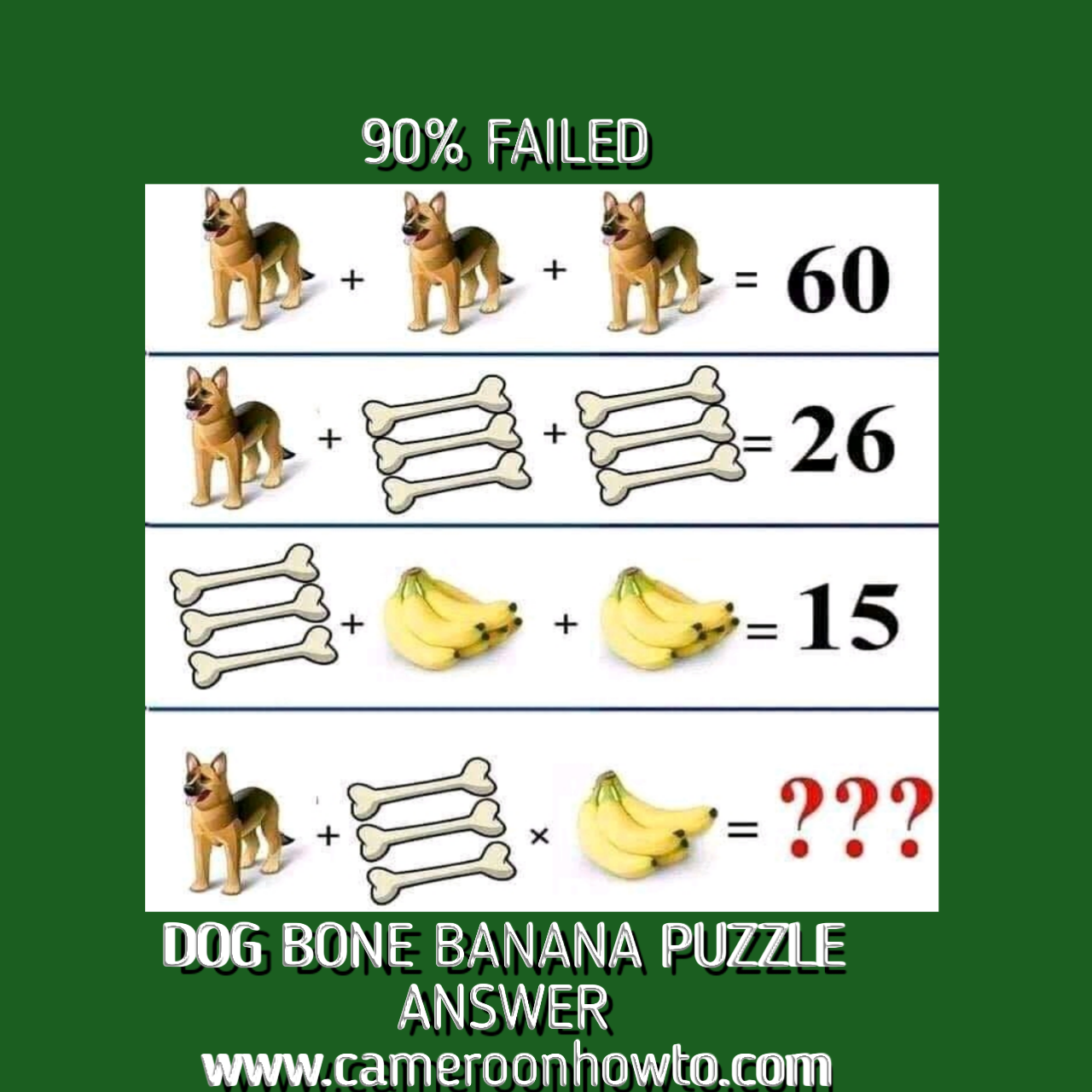0

# Answer to dog bone banana math puzzleDog Bone banana Puzzle
Welcome to www.cammeroonhowto.com where I solve tricky math puzzles, quizzes, riddles and more with detailed explanations. Today I will solve the " dog bone banana Puzzle for you.

This is one of those tricky math  puzzles that keeps many people scratching their heads on the internet, especially on Facebook.  When the Puzzle was published on Facebook, many people solved it and had different opinions and answers. Solving Math Puzzles can be interesting and funny. Be sure to share this one with loved ones.

Dog + bone × bananas = ?

The correct answer would be 32 not 92, the trick in the math Puzzle is found in the last equation because the bunch of bananas has four bananas meanwhile that in the third equation has 5 bananas. Another trick is to multiply before adding.

Many game lovers like solving math puzzles suduku, quizzes like this one to refresh their brain.

Here's the puzzle extraction:

If

Dog + dog + dog  = 60,

Dog + 3bones + 3bones = 26

Bones +5 bananas + 5 bananas = 15

then,

Dog + bones × four bananas = ?

Due to the Corona various pandemic, many people isolate themselves because of lockdowns and to avoid contracting or spreading the deadly disease.

Isolation can lead to boredom,  one way to refresh the brain, relax and get busy is  by solving Math puzzles and Quizzes.

I will form four equations, solve equation 1, 2 and three mathematically and then substitute answers in the final equation and simplify.

Equation one

3dogs =60

Implies dog   = 60÷3,

Dog = 20.

Equation two

20 + 6bones = 26

Implies 6bones = 26 minus 20

6bones = 6

Bone = 6 ÷ 6

Bone  = 1

Equation three

3 + 6 banana + 6 banana = 15,

12 banana = 15 minus 3

12 banana = 12

Banana = 12/12=  1.

Therefore, the value of Dog Bone and banana are as Thus:

Dog = 20

Bone = 1

Banana = 1

Remember you can still get all the values by simply counting but it's important to use math rules in case of larger numbers.

Final equation

I will substitute the values of the variables above in the final equation below and simplify.

Dog + 3 bone × 4 bananas = ?

20 + 3 × 4 = ? ( Apply BODMAS)

20 + 12= ?

32 = ?

So the correct answer to the tricky  math Puzzle "dog + 3bones × 4 bananas =?"  Is

= 32.

If you don't apply BODMAS, you will get 92 which is a mathematically wrong answer.

Do you have another way to arrive at this Answer? Share with us.

Don't forget to test your friends, family members and loved ones by sharing the puzzle.